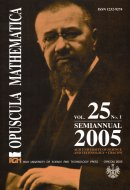Opuscula Math. 25, no. 1 (2005), 149-160

Opuscula Mathematica

# On some application of biorthogonal spline systems to integral equations

Zygmunt Wronicz

Abstract. We consider an operator $$P_N: L_p(I) \to S_n(\Delta_N)$$, such that $$P_Nf=f$$ for $$f\in S_n(\Delta_N)$$, where $$S_n(\Delta_N)$$ is the space of splines of degree $$n$$ with respect to a given partition $$\Delta_N$$ of the interval $$I$$. This operator is defined by means of a system of step functions biorthogonal to $$B$$-splines. Then we use this operator to approximation to the solution of the Fredholm integral equation of the second kind. Convergence rates for the approximation of the solution of this equation are given.

Keywords: operator associated with step functions, $$B$$-splines, integral equation, approximation.

Mathematics Subject Classification: 41A15, 45B05, 45L10, 65R20.

Full text (pdf)

• Zygmunt Wronicz
• AGH University of Science and Technology, Faculty of Applied Mathematics, al. Mickiewicza 30, 30-059 Cracow, Poland